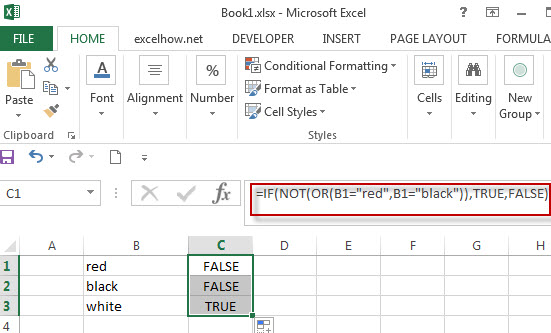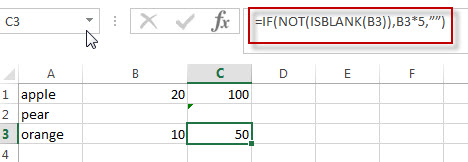# Excel IF formula with NOT logical function

This post will guide you how to use excel if formula with NOT logical function in Microsoft excel.

## NOT Logical Function

The syntax of NOT logical function is as follow:

`=NOT(logical_value)`

The NOT logical function will return the reversed logical value. If the logical_value is TRUE, then FALSE is returned. If the logical_value is FALSE, then TRUE is returned. Let’s see the below two examples:

```=NOT(FALSE)
=NOT(2+1=3)```

Both of the above excel formulas will return TRUE.

## Excel IF Formula Combining With NOT

If you want to check several test conditions in an excel formula,then take different actions. You can use NOT function in combination with the AND or OR logical function in excel IF function.

Let’s see the following excel if formula:

`=IF(NOT(OR(B1="red",B1="black")),TRUE,FALSE)`

In the above formula, the excel formula will exclude both red and blank colors. It will check cells in column B if the values is NOT red or blank. TRUE or FALSE will be returned.Actually, we also can use the Not equal to (<>) logical operator to achieve the same requirement , For example, we can write down the following excel IF formula to reflect the above logic test.

`=IF(AND(B1<>”red”,B1<>”black”)),TRUE,FALSE)`

## NOT Function combining with ISBLANK function

As you know, the function ISBLANK(B1) will return TRUE if the cell B1 is blank. If we use NOT function to enclose ISBLANK(B1) function, it will reverse the result, if the cell is blank, then returns FALSE.

Let’s create a nested IF statement with the NOT and ISBLANK functions as follow:

`=IF(NOT(ISBLANK(B1)),B1*5,””)`For above IF statement, IF the cell B1 is not blank, then multiply the number in B1 by 5.we can enter the above formula into cell C1, and then drag the Fill Handle to the range C1:C3.it will apply for the other cells for this formula.

### Related Functions

• Excel OR function
The Excel OR function returns TRUE if any of the conditions are TRUE in logic test. Otherwise, it returns FALSE.
• Excel AND function
The Excel AND function returns TRUE if all of arguments are TRUE, and it returns FALSE if any of arguments are FALSE.The syntax of the AND function is as below:= AND (condition1,[condition2],…) …
• Excel IF function
The Excel IF function perform a logical test to return one value if the condition is TRUE and return another value if the condition is FALSE….
• Excel nested if function
The nested IF function is formed by multiple if statements within one Excel if function. This excel nested if statement makes it possible for a single formula to take multiple actions…
• Excel ISBLANK function
The Excel ISBLANK function returns TRUE if the value is blank or null.The syntax of the ISBLANK function is as below:= ISBLANK (value)…
• Excel NOT function
The Excel NOT function returns the opposite of a given logical or Boolean value. For example, if you supplied with the value TRUE, the NOT function will return FALSE; If you supplied with the value FALSE, and the NOT function will TRUE. The syntax of the NOT function is as below:=NOT(logical)…

Related Posts

VLOOKUP Formula | Faster Trick with 2 VLOOKUPS

This post will guide you how to use 2 VLOOKUPS function to looking up data entries from a given range of cells in Microsoft Excel. VLOOKUP with 2 lookups can be faster than a single VLOOKUP in certain scenarios. The ...

Extract all the matches with helper Column

With Excel's powerful functions IF, INDEX, and MATCH, we can find exactly what you're looking for with a few clicks of the mouse. This step-by-step tutorial will show how easy it is to extract data using these tools and more! ...

If Cell is This Value or That Value

IF function is frequently used in Excel worksheet to return you expect “true value” or “false value” based on the result of logical test. If you want to see if a cell is A or B, and if one of ...

If Value is Greater Than A Certain Value

IF function is frequently used in Excel worksheet to return you expect “true value” or “false value” based on the logical test result. If you want to see if a value in one cell is greater than a specific value, ...

If Cell is Not Blank

IF function is frequently used in Excel worksheet to return you expect “true value” or “false value” based on the result of created logical test. If you want to see if a cell is blank or not, and leave some ...

Sidebar